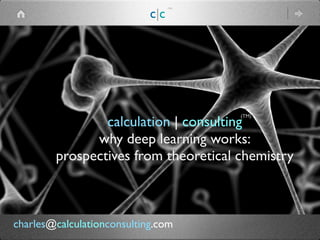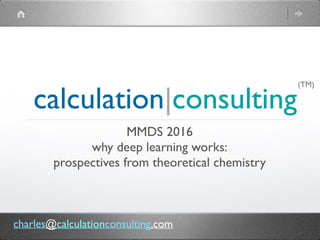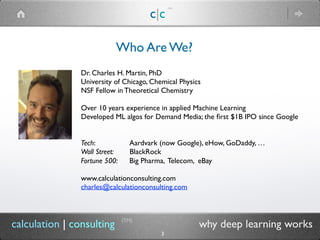Successfully reported this slideshow.

# CC mmds talk 2106

16

Share×
1 of 45
1 of 45

# CC mmds talk 2106

16

Share

Why Deep Learning Works: Perspectives from Theoretical Chemistry

Why Deep Learning Works: Perspectives from Theoretical Chemistry

## More Related Content

### Related Books

Free with a 14 day trial from Scribd

See all

### Related Audiobooks

Free with a 14 day trial from Scribd

See all

### CC mmds talk 2106

1. 1. calculation | consulting why deep learning works: perspectives from theoretical chemistry (TM) c|c (TM) charles@calculationconsulting.com
2. 2. calculation|consulting MMDS 2016 why deep learning works: perspectives from theoretical chemistry (TM) charles@calculationconsulting.com
3. 3. calculation | consulting why deep learning works Who Are We? c|c (TM) Dr. Charles H. Martin, PhD University of Chicago, Chemical Physics NSF Fellow in Theoretical Chemistry Over 10 years experience in applied Machine Learning Developed ML algos for Demand Media; the ﬁrst \$1B IPO since Google Tech: Aardvark (now Google), eHow, GoDaddy, … Wall Street: BlackRock Fortune 500: Big Pharma, Telecom, eBay www.calculationconsulting.com charles@calculationconsulting.com (TM) 3
4. 4. Data Scientists are Different c|c (TM) theoretical physics machine learning specialist (TM) 4 experimental physics data scientist engineer software, browser tech, dev ops, … not all techies are the same calculation | consulting why deep learning works
5. 5. c|c (TM) Problem: How can SGD possibly work? Aren’t Neural Nets non-Convex ?! (TM) 5 calculation | consulting why deep learning works can Spin Glass models suggest why ? what other models are out there ? expected observed ?
6. 6. c|c (TM) (TM) 6 calculation | consulting why deep learning works Outline  Random Energy Model (REM) Temperature, regularization and the glass transition extending REM: Spin Glass of Minimal Frustration protein folding analogy: Funneled Energy Landscapes example: Dark Knowledge Recent work: Spin Glass models for Deep Nets
7. 7. c|c (TM) (TM) 7 calculation | consulting why deep learning works Warning  condensed matter theory is about qualitative analogies we may seek a toy model a mean ﬁeld theory a phenomenological description
8. 8. c|c (TM) What problem is Deep Learning solving ? (TM) 8 calculation | consulting why deep learning works minimize cross-entropy https://www.ics.uci.edu/~pjsados.pdf
9. 9. c|c (TM) Problem: What is a good theoretical model for deep networks ? (TM) 9 calculation | consulting why deep learning works p-spin spherical glass LeCun … 2015 L Hamiltonian (Energy function) X Gaussian random variables w real valued (spins) , spherical constraint H >= 3 (p) can be solved analytically, simulated easily
10. 10. c|c (TM) What is a spin glass ? (TM) 10 calculation | consulting why deep learning works Frustration: constraints that can not be satisﬁed J = X = weights S = w = spins Energetically: all spins should be paired
11. 11. c|c (TM) why p-spin spherical glass ? (TM) 11 calculation | consulting why deep learning works crudely: deep networks (effectively) have no local minima ! local minima k=1 critical points ﬂoor / ground state k = 2 critical points k = 3 critical points the critical points are ordered saddle points
12. 12. c|c (TM) why p-spin spherical glass ? (TM) 12 calculation | consulting why deep learning works crudely: deep networks (effectively) have no local minima ! http://cims.nyu.edu/~achoroma/NonFlash/Papers/PAPER_AMMGY.pdf ap
13. 13. c|c (TM) (TM) 13 calculation | consulting why deep learning works any local minima will do; the ground state is a state of overtraining good generalization overtraining Early Stopping: to avoid the ground state ?
14. 14. c|c (TM) (TM) 14 calculation | consulting why deep learning works it’s easy to ﬁnd the ground state; it’s hard to generalize ? Early Stopping: to avoid the ground state ?
15. 15. c|c (TM) Current Interpretation  (TM) 15 calculation | consulting why deep learning works •ﬁnding the ground state is easy (sic); generalizing is hard •ﬁnding the ground state is irrelevant: any local minima will do •the ground state is a state over training
16. 16. c|c (TM) recent p-spin spherical glass results (TM) 16 calculation | consulting why deep learning works actually: recent results (2013) on the behavior (distribution of critical points, concentration of the means) of an isotropic random function on a high dimensional manifold require: the variables actually concentrate on their means the weights are drawn from isotropic random function related to: old results TAP solutions (1977) # critical points ~ TAP complexity avoid local minima? : increase Temperature harder problem: low Temp behavior of spin glass
17. 17. c|c (TM) What problem is Deep Learning solving ? (TM) 17 calculation | consulting why deep learning works minimize cross-entropy of output layer entropic effects : not just min energy more like min free energy (divergence) Statistical Physics and InformationTheory: Neri Merhav i.e. variational auto encoders
18. 18. c|c (TM) What problem is Deep Learning solving ? (TM) 18 calculation | consulting why deep learning works Restricted Boltzmann Machine can deﬁne free energy directly A Practical Guide toTraining Restricted Boltzmann Machines, Hinton
19. 19. c|c (TM) What problem is Deep Learning solving ? (TM) 19 calculation | consulting why deep learning works Restricted Boltzmann Machine trade off between energy and entropy min free energy directly A Practical Guide toTraining Restricted Boltzmann Machines, Hinton
20. 20. c|c (TM) (TM) 20 calculation | consulting why deep learning works https://web.stanford.edu/~montanar/RESEARCH/BOOK/partB.pdf inﬁnite limit of p-spin spherical glass A related approach: Random Energy Model (REM)
21. 21. c|c (TM) Random Energy Model (REM) (TM) 21 calculation | consulting why deep learning works ground state is governed by ExtremeValue Statistics http://guava.physics.uiuc.edu/~nigel/courses/563/essays2000/pogorelov.pdf http://scitation.aip.org/content/aip/journal/jcp/111/14/10.1063/1.479951 old result from protein folding theory
22. 22. c|c (TM) REM: What is Temperature ? (TM) 22 calculation | consulting why deep learning works We can use statistical mechanics to analyze known algorithms I don’t mean in the traditional sense of algorithmic analysis take Ej as the objective = loss function + regularizer study Z: form a mean ﬁeld theory; take limits N -> inf, T -> 0
23. 23. c|c (TM) REM: What is Temperature ? (TM) 23 calculation | consulting why deep learning works let E(T) by the effective energy E(T) = E/T ~ sum of weights*activations as T -> 0, E(T) effective energies diverge; weights explode Temperature is a proxy for weight constraints T sets the Energy Scale
24. 24. c|c (TM) Temperature: as Weight Constraints (TM) 24 calculation | consulting why deep learning works •traditional weight regularization •max norm constraints (i.e. w/dropout) •batch norm regularization (2015) we avoid situations when the weights explode in deep networks, we temper the weights and the distribution of the activations (i.e local entropy)
25. 25. c|c (TM) REM: a toy model for real Glasses  (TM) 25 calculation | consulting why deep learning works but it is believed that entropy collapse ‘drives’ the glass transition the glass transition is not well understood
26. 26. c|c (TM) what is a real (structural) Glass ?  (TM) 26 calculation | consulting why deep learning works Sand + Fire = Glass
27. 27. c|c (TM) what is a real (structural) Glass ?  (TM) 27 calculation | consulting why deep learning works all liquids can be made into glasses if we cool then fast enough the glass transition is not a normal phase transition not the melting point arrangement of atoms is amorphous; not completely random different cooling rates produce different glassy states universal phenomena; not universal physics molecular details affect the thermodynamics
28. 28. c|c (TM) REM: the Glass Transition  (TM) 28 calculation | consulting why deep learning works Entropy collapses when T <~ Tc Phase Diagram: entropy density energy density free energy density https://web.stanford.edu/~montanar/RESEARCH/BOOK/partB.pdf
29. 29. c|c (TM) REM: Dynamics on the Energy Landscape  (TM) 29 calculation | consulting why deep learning works let us assume some states trap the solver for some time; of course, there is a great effort to design solvers that can avoid traps
30. 30. c|c (TM) Energy Landscapes: and Protein Folding   (TM) 30 calculation | consulting why deep learning works let us assume some states trap the solver in state E(j) for a short time and the transitions E(j) -> E(j-1) are governed by ﬁnite, reversible transitions (i.e. SGD oscillates back and forth for a while) classic result(s): for T near the glass Temp (Tc) the traversal times are slower than exponential ! in a physical system, like a protein or polymer, it would take longer than the known lifetime of the universe to ﬁnd the ground (folded) state
31. 31. c|c (TM) Protein Folding: the Levinthal Paradox   (TM) 31 calculation | consulting why deep learning works folding could take longer than the known lifetime of the universe ?
32. 32. c|c (TM) (TM) 32 calculation | consulting why deep learning works http://arxiv.org/pdf/cond-mat/9904060v2.pdf Old analogy between Protein folding and Hopﬁeld Associative Memories Natural pattern recognition could • use a mechanism with a glass Temp (Tc) that is as low as possible • avoid the glass transition entirely, via energetics Nature (i.e. folding) can not operate this way ! Protein Folding: around the Levinthal Paradox
33. 33. c|c (TM) Spin Glasses: Minimizing Frustration  (TM) 33 calculation | consulting why deep learning works http://www.nature.com/nsmb/journal/v4/n11/pdf/nsb1197-871.pdf
34. 34. c|c (TM) Spin Glasses: Minimizing Frustration  (TM) 34 calculation | consulting why deep learning works http://www.nature.com/nsmb/journal/v4/n11/pdf/nsb1197-871.pdf
35. 35. c|c (TM) Spin Glasses: vs Disordered FerroMagnets  (TM) 35 calculation | consulting why deep learning works http://arxiv.org/pdf/cond-mat/9904060v2.pdf
36. 36. c|c (TM) the Spin Glass of Minimal Frustration   (TM) 36 calculation | consulting why deep learning works REM + strongly correlated ground state = no glass transition https://arxiv.org/pdf/1312.7283.pdf
37. 37. c|c (TM) the Spin Glass of Minimal Frustration   (TM) 37 calculation | consulting why deep learning works Training a model induces an energy gap, with few local minima http://arxiv.org/pdf/1312.0867v1.pdf
38. 38. c|c (TM) Energy Funnels: Entropy vs Energy   (TM) 38 calculation | consulting why deep learning works there is a tradeoff between Energy and Entropy minimization
39. 39. c|c (TM) Energy Landscape Theory of Protein Folding (TM) 39 calculation | consulting why deep learning works there is a tradeoff between Energy and Entropy minimization
40. 40. c|c (TM) (TM) 40 calculation | consulting why deep learning works Avoids the glass transition by having more favorable energetics Levinthal paradox glassy surface vanishing gradients Energy Landscape Theory of Protein Folding funneled landscape rugged convexity energy / entropy tradeoff
41. 41. c|c (TM) RBMs: Entropy Energy Tradeoff (TM) 41 calculation | consulting why deep learning works RBM on MNIST Aligned for comparison: entropy - 200 Entropy drops off much faster than total Free Energy
42. 42. c|c (TM) Dark Knowledge: an Energy Funnel ?  (TM) 42 calculation | consulting why deep learning works 784 -> 800 -> 800 -> 10MLP on MNIST Distilled 10,000 test cases, 10 classes 99 errors same entropy (capacity); better loss function ﬁt to ensemble soft-max probabilities 146 errors 784 -> 800 -> 800 -> 10
43. 43. c|c (TM) Adversarial Deep Nets: an Energy Funnel ?  (TM) 43 calculation | consulting why deep learning works Discriminator learns a complex loss function Generator: fake data Discriminator: fake vs real ? http://soumith.ch/eyescream/
44. 44. c|c (TM) (TM) 44 calculation | consulting why deep learning works Summary  Random Energy Model (REM): simpler theoretical model Glass Transition: temperature ~ weight constraints extending REM: Spin Glass of Minimal Frustration possible examples: Dark Knowledge Funneled Energy Landscapes Adversarial Deep Nets
45. 45. (TM) c|c (TM) c | c charles@calculationconsulting.com